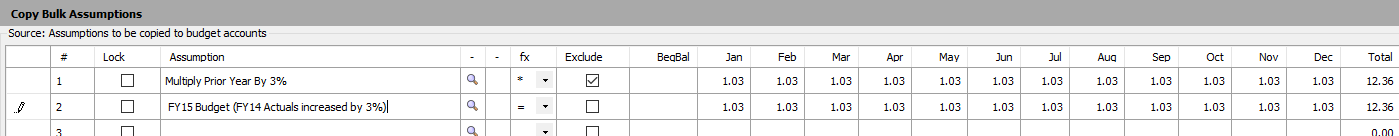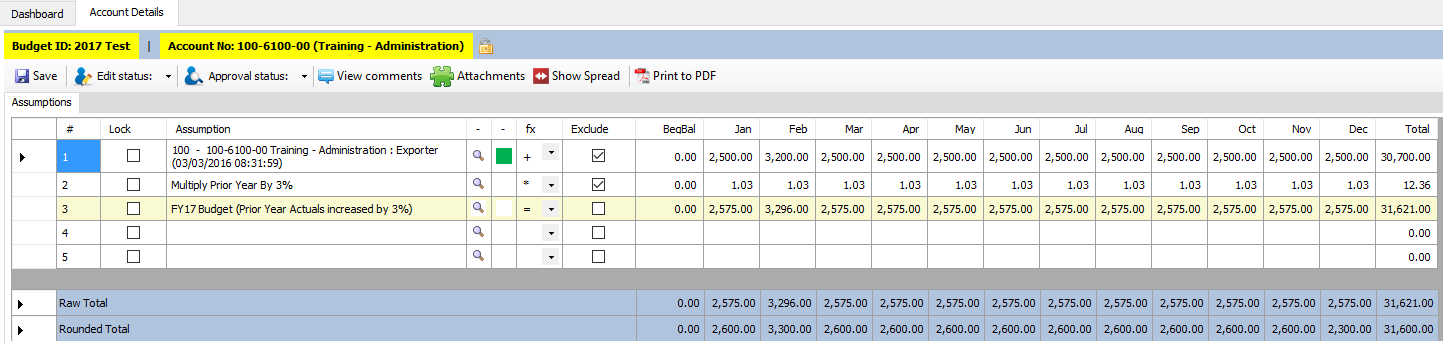This process requires the use of the Import Budget Assumptions and Copy Bulk Assumptions

The budget template you wish to populate will need to have been setup and listed in Manage Budgets as will have the required budget account numbers.

Please see How Do I Setup a Budget (including for the New Year)? to create a budget template.

The required result on the Account Details screen is as follows:

Row 1: Prior Year Actuals Data

Row 2: Increase Row 1 by 3 percent

Row 3: The result from the Row 1 and Row 2 calculation.

1) First use the Import Budget Assumptions tool to perform a Lookup using the "Financial" tab for the required prior year actuals data and import that data as Row 1 for the required budget account/s. The import data should be marked as "Excluded".  See Row 1 below.2) On Copy Bulk Assumptions using the Exclude row and Row to Row Calculations setup the above Row 2 (the 3% increase) and Row 3 (the required budget)  as per below.

Please note that the Row 2 (the inflation factor ) could be setup as a Global Variables in Manage Global Variables and accessed through Lookup.  The advantage could be that when the Global Variable Inflation Factor is updated, all the linked Account totals are automatically updated.  Please see How Can I Use Global Variables?*Note the row with the math operator (fx) "=" will not preview correctly on screen as it has no context without the first row of the calculation to know how to complete the formula. Though it appears to only duplicate the line above it, the final result will be correct.

When the Copy Bulk Assumptions process is performed, the incomplete 2 line equation will be inserted as the next 2 rows in each of the destination accounts (as below - Row 2 and 3).  The "save" routine will recompute the row over row math formulas for each account and save the results. As per Row 3 below the final result would have appeared with unique calculated amount for each respective account.*Reminder Tip: For Row over Row Math Formulas, all lines except for the final subtotal should be marked as Excluded. Unmarked rows will be included in the total for the account.